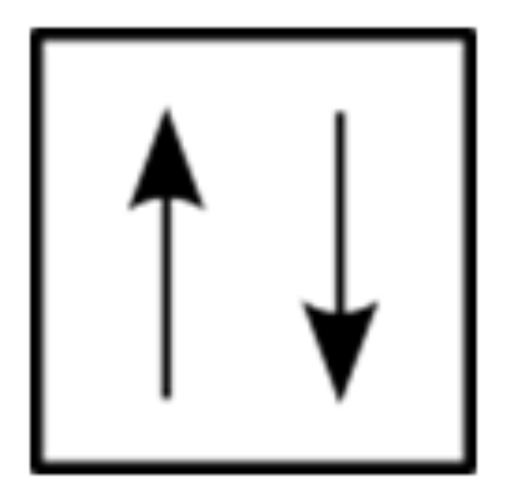Cyber Monday Sale: ALL MCAT Products & Admissions Services

MCAT Content / Electronic Structure / Use Of Pauli Exclusion Principle

### Use of Pauli Exclusion Principle

Topic: Electronic Structure

The Pauli exclusion principle states that no two electrons in an atom can have the same set of four quantum numbers.

The Pauli exclusion principle can be applied to all quantum numbers, which states that no two electrons in an atom can have the same set of four quantum numbers. The four quantum numbers need to unique.

Since no two electrons can have the same set of quantum numbers, there are limits to how many of them can be in the same energy state. Note that n determines the energy state in the absence of a magnetic field.

Consider the n=1 level, for example. The only value l can have 0, and thus ml can only be 0. The spin projection ms can be either +1/2 or −1/2, and so there can be two electrons in the = 1 state.This is represented in an orbital filling diagram, a square represents an orbital, while arrows represent electrons. An arrow pointing upward represents one spin direction, while an arrow pointing downward represents the other spin direction.

Because of the Pauli exclusion principle, only hydrogen and helium can have all of their electrons in the = 1 state. Lithium (see the periodic table) has three electrons, and so one must be in the = 2 level. This leads to the concept of shells and shell filling. As we progress up in the number of electrons, we go from hydrogen to helium, lithium, beryllium, boron, and so on, and we see that there are limits to the number of electrons for each value of n. Higher values of the shell n correspond to higher energies, and they can allow more electrons because of the various combinations of lml, and ms that are possible. Each value of the principal quantum number n thus corresponds to an atomic shell into which a limited number of electrons can go. Shells and the number of electrons in them determine the physical and chemical properties of atoms since it is the outermost electrons that interact most with anything outside the atom.

Practice Questions

Imaging tissue structures using muon tomography

MCAT Official Prep (AAMC)

Key Points

• No two electrons in an atom can have the same set of four quantum numbers.

• If the energy (n), angular momentum (ℓ), magnetic moment (mℓ) numbers are identical for two electrons, the spin numbers must be different.

Key Terms

atom: The smallest possible amount of matter that still retains its identity as a chemical element. Atoms consist of a nucleus surrounded by electrons.

orbital: physical region or space where the electron can be calculated to be present.

quantum numbers: used to describe characteristics of electrons

electron: each electron has a negative charge (-1) with weight so small it’s normally negligible as compared to proton or neutron.

Billing Information